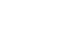Fourth quarterly report of PAS in 2018

In the fourth quarter of 2018, the total revenue of PAS is KHR 75,441,319,000 which increases by KHR 12,598,319,000 equal to 20.05% and the net profit is KHR 12,744,214,000 which increases by KHR 1,119,947,000 equal to 9.63% if compared to the same period of 2017. At the end of 31st December 2018, total revenue is KHR 275,575,645,000 which increases by KHR 48,177,810,000 equal to 21.19% and net profit is KHR 42,236,172,000 which increases by KHR 17,123,856,000 equal to 68.12% if compared to the same period of 2017. At the end of 31st December 2018, total asset is KHR 1,328,190,488,000 which increases by KHR 47,680,450,000 equal to 3.72% and total equity is KHR 670,411,834,000 which increases by KHR 23,801,280,000 equal to 3.68%

Fourth quarterly report of PAS in 2018

In the fourth quarter of 2018, the total revenue of PAS is KHR 75,441,319,000 which increases by KHR 12,598,319,000 equal to 20.05% and the net profit is KHR 12,744,214,000 which increases by KHR 1,119,947,000 equal to 9.63% if compared to the same period of 2017. At the end of 31st December 2018, total revenue is KHR 275,575,645,000 which increases by KHR 48,177,810,000 equal to 21.19% and net profit is KHR 42,236,172,000 which increases by KHR 17,123,856,000 equal to 68.12% if compared to the same period of 2017. At the end of 31st December 2018, total asset is KHR 1,328,190,488,000 which increases by KHR 47,680,450,000 equal to 3.72% and total equity is KHR 670,411,834,000 which increases by KHR 23,801,280,000 equal to 3.68%.

The significant indicators in the fourth quarter of 2018 are as follows:

1. Current ratio: 3.33
2. Quick ratio: 3.13
3. Return on asset: 0.96%
4. Return on Equity: 1.90%
5. Gross profit margin: 25.53%
6. Profit margin: 16.89%
7. Basic earnings per share: KHR 148.58

Please refer to the attached file for more details: